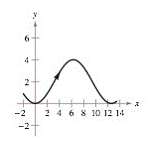Chapter 10.3, Problem 28E

Chapter
Section
Textbook Problem

# Horizontal and Vertical Tangency In Exercises 31 and 32, find all points (if any) of horizontal and vertical tangency to the curve on the given interval. x = 2 θ y = 2 ( 1 − cos θ ) 0 ≤ θ ≤ 2 πTo determine

All the points of horizontal & vertical tangency to the curve on given interval.

Explanation

Given:

The provided figure is,

x=2θ&y=2(1cosθ) & 0θ2π

Calculation:

Differentiate both the functions with respect to θ,

dxdθ=2 & dydθ=2sinθ

For vertical tangency equate dxdθ=2

### Still sussing out bartleby?

Check out a sample textbook solution.

See a sample solution

#### The Solution to Your Study Problems

Bartleby provides explanations to thousands of textbook problems written by our experts, many with advanced degrees!

Get Started